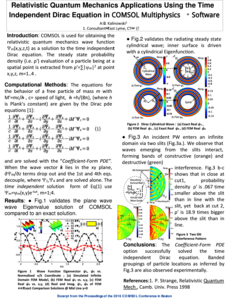# 论文和技术资料

## Relativistic Quantum Mechanics Applications Using the Time Independent Dirac Equation in COMSOL Multiphysics® Software

A. J. Kalinowski ,
 Consultant, East Lyme, CT, USA

The paper illustrates the use of the COMSOL Multiphysics® software (via the “Coefficient-Form PDE” option) for obtaining the relativistic quantum mechanics wave function ψm(x,y,ω), m=1,4 as a solution to the time independent Dirac equations. Once having ψm(x,y,ω), it can be used to compute the probability density ρ′=Σ|ψm|^2 of a particle being at a specific point within the spatial field under consideration. Four problems are solved to illustrate solving Dirac equations, the first three being validation through FEM comparison to closed form exact solutions, and the last example is for a plane wave impinging onto two slit barrier.